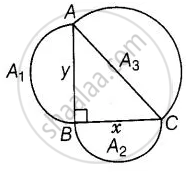# Prove that the area of the semicircle drawn on the hypotenuse of a right angled triangle is equal to the sum of the areas of the semicircles drawn on the other two sides of the triangle. - Mathematics

Sum

Prove that the area of the semicircle drawn on the hypotenuse of a right-angled triangle is equal to the sum of the areas of the semicircles drawn on the other two sides of the triangle.

#### Solution

Let ABC be a right triangle, right-angled at B and AB = y, BC = x.

Three semi-circles are drawn on the sides AB, BC and AC, respectively with diameters AB, BC and AC, respectively.

Again, let area of circles with diameters AB, BC and AC are respectively A1, A2 and A3.To prove: A_3 = A_1 + A_2

Proof: In ΔABC, by Pythagoras theorem,

AC^2 = AB^2 + BC^2

⇒ AC^2 = y^2 + x^2

⇒ AC = sqrt(y^2 + x^2)

We know that, area of a semi-circle with radius, r = (pir^2)/2

∴ Area of semi-circle drawn on AC, A_3 = pi/2((AC)/2)^2 = pi/2(sqrt(y^2 + x^2)/2)^2

⇒ A_3 = (pi(y^2 + x^2))/8  .......(i)

Now, area of semi-circle drawn on AB, A_1 = pi/2 ((AB)/2)^2

⇒ A_1 = pi/2(y/2)^2

⇒ A_1 = (piy^2)/8  ......(ii)

And area of semi-circle drawn on BC, A_2 = pi/2((BC)/2)^2 = pi/2(x/2)^2

⇒ A_2 = (pix^2)/8

On adding equations (ii) and (ii), we get

A_1 + A_2 = (piy^2)/8 + (pix^2)/8

= (pi(y^2 + x^2))/8

= A_3   .....[From equation (i)]

⇒ A1 + A2 = A3

Hence proved.

Concept: Application of Pythagoras Theorem in Acute Angle and Obtuse Angle
Is there an error in this question or solution?

#### APPEARS IN

NCERT Mathematics Exemplar Class 10
Chapter 6 Triangles
Exercise 6.4 | Q 17 | Page 76

Share